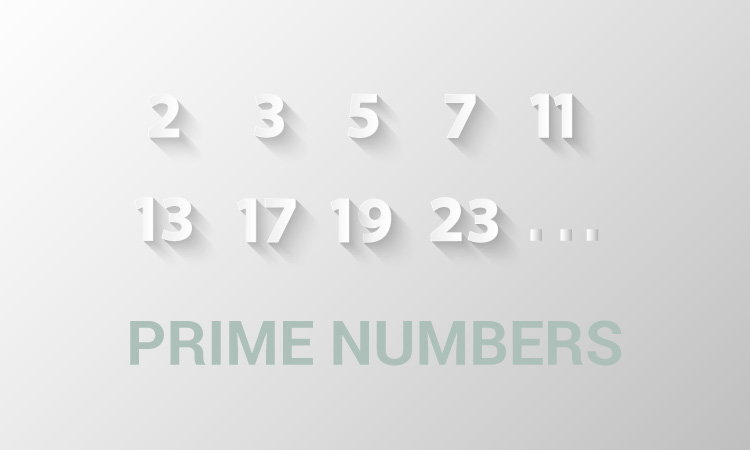# C Program to Check Whether a Number is Prime or Not

Example to check whether an integer (entered by the user) is a prime number or not using for loop and if...else statement.To understand this example, you should have the knowledge of following C programming topics:

A prime number is a positive integer which is divisible only by 1 and itself. For example: 2, 3, 5, 7, 11, 13

## Example: Program to Check Prime Number

``````#include <stdio.h>
int main()
{
int n, i, flag = 0;

printf("Enter a positive integer: ");
scanf("%d", &n);

for(i = 2; i <= n/2; ++i)
{
// condition for nonprime number
if(n%i == 0)
{
flag = 1;
break;
}
}

if (n == 1)
{
printf("1 is neither a prime nor a composite number.");
}
else
{
if (flag == 0)
printf("%d is a prime number.", n);
else
printf("%d is not a prime number.", n);
}

return 0;
}``````

Output

```Enter a positive integer: 29
29 is a prime number.```

If the `for` loop terminates when the test expression of loop `i <= n/2` is false, the entered number is a prime number. The value of flag is equal to 0 in this case.

If the loop terminates because of `break` statement inside the `if` statement, the entered number is a nonprime number. The value of flag is 1 in this case.

Visit this page to learn, how you can display all prime numbers between two intervals entered by the user.